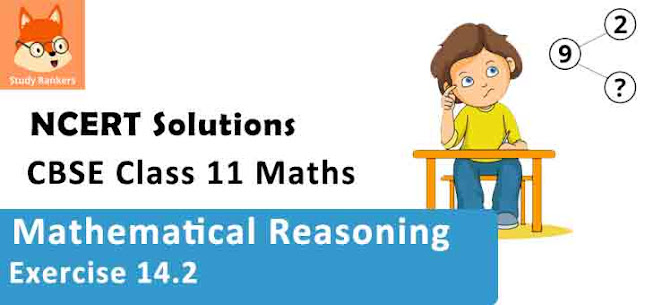# Class 11 Maths NCERT Solutions for Chapter 14 Mathematical Reasoning Exercise 14.2### Mathematical Reasoning Exercise 14.2 Solutions

1. Write the negation of the following statements:
(i) Chennai is the capital of Tamil Nadu.
(ii) √2 is not a complex number.
(iii) All triangles are not equilateral triangle.
(iv) The number 2 is greater than 7.
(v) Every natural number is an integer.

Solution

(i) Chennai is not the capital of Tamil Nadu.
(ii) √2 is a complex number.
(iii) All triangles are equilateral triangles.
(iv) The number 2 is not greater than 7.
(v) Every natural number is not an integer.

2. Are the following pairs of statements negations of each other?
(i) The number x is not a rational number.
The number x is not an irrational number.
(ii) The number x is a rational number.
The number x is an irrational number.

Solution

(i) The negation of the first statement is “the number x is a rational number”.
This is same as the second statement. This is because if a number is not an irrational number, then it is a rational number.
Therefore, the given statements are negations of each other.

(ii) The negation of the first statement is “the number x is not a rational number”. This means that the number x is an irrational number, which is the same as the second statement.
Therefore, the given statements are negations of each other.

3. Find the component statements of the following compound statements and check whether they are true or false.
(i) Number 3 is prime or it is odd.
(ii) All integers are positive or negative.
(iii) 100 is divisible by 3, 11 and 5.

Solution

(i) The component statements are as follows.
p: Number 3 is prime.
q: Number 3 is odd.
Both the statements are true.

(ii) The component statements are as follows.
p: All integers are positive.
q: All integers are negative.
Both the statements are false.

(iii) The component statements are as follows.
p: 100 is divisible by 3.
q: 100 is divisible by 11.
r: 100 is divisible by 5.
Here, the statements, p and q, are false and statement r is true.